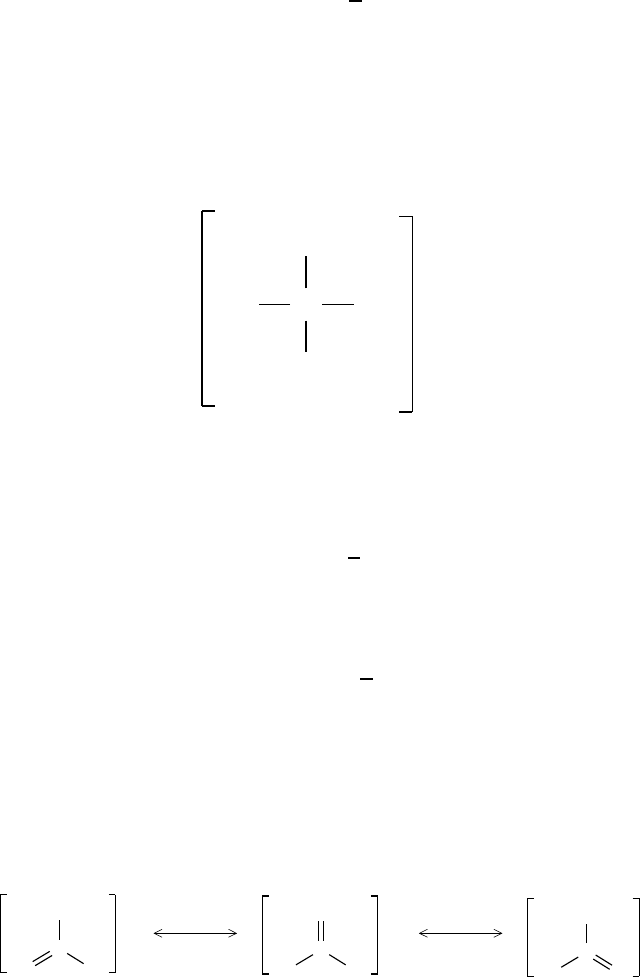Textbook Notes (290,000)
CA (170,000)
Queen's (4,000)
CHEM (40)
Chapter

# CHEM 112 Chapter Notes -Formal Charge, Lewis Structure, Covalent Bond

This preview shows pages 1-2. to view the full 6 pages of the document.Formal and partial charges
Marc R. Roussel
Department of Chemistry and Biochemistry
University of Lethbridge
October 24, 2005
1 Formal charge
In an ideal covalent bond, electrons are exactly equally shared. Thus, we can
imagine that half the electrons in each bond “live” on each of the two atoms
participating in that bond at any given time. This leads to a very simple
formula for the charge carried by an atom: We can easily determine the
number of valence electrons of a neutral atom. If we can ﬁgure out whether
an atom in a molecule has more or less valence electrons than the neutral
atom, then we immediately know its charge. In other words, the diﬀerence
between the number of valence electrons of the neutral atom and the number
of electrons it carries in a molecule is an estimate of its charge:
Qf=VL+1
2B,(1)
where Qfis the formal charge,Vis the number of valence electrons of the
neutral atom, Lis the number of electrons in lone pairs (not the number of
lone pairs), and Bis the number of electrons in covalent bonds to the atom.
Note that the sum of the formal charges in a molecular species is equal to
the total charge, zero for a neutral molecule.
Example 1.1 The Lewis diagram of carbon dioxide is
C OO ..
....
..
1

Only pages 1-2 are available for preview. Some parts have been intentionally blurred.For carbon, V= 4. In this molecule, the carbon atom has L= 0 and
B= 8. The formal charge is therefore
Qf(C) = 4 1
2(8) = 0.
The formal charges on the oxygen atoms are also zero.
Example 1.2 The Lewis diagram of the sulfate ion is
S
:O:
:O:
:O O:
2−
..
..
..
....
..
For sulfur, V= 6. In this ion, the sulfur atom has L= 0 and B= 8.
The formal charge is therefore
Qf(S) = 6 1
2(8) = 2.
For the oxygens, we have V= 6, L= 6 and B= 2, which gives
Qf(O) = 6 6 + 1
2(2)=1.
Note Qf(S) + 4Qf(O) =2, the charge on the ion.
Example 1.3 The carbonate ion has resonance structures:
C
:O:
:O O:
C
:O:
:O O: C
:O:
:O O:
2−
..
..
.. ..
2− 2−
.. ..
..
..
..
.. ..
..
2